Disclaimer: This is an example of a student written essay.

Any scientific information contained within this essay should not be treated as fact, this content is to be used for educational purposes only and may contain factual inaccuracies or be out of date.

 ✅ Paper Type: Free Essay ✅ Subject: Chemistry ✅ Wordcount: 3563 words ✅ Published: 30th Jan 2018

List of Apparatus

 Name of Apparatus Quantity Uncertainty Electronic Stopwatch 1 ±0.2s Ammeter 1 ±0.01A Wires with crocodile clips 1 – D.C power source 1 ±0.01V 300cm3 Beaker 3 – Copper strips 2 – Sand paper 1 – Graphite rod 2 – pH probe/data-logger 1 ±0.2 Electronic weighing balance 1 ±0.001

DATA COLLECTION:

The electrolytic cell used in this investigation is illustrated in Fig 1: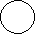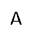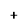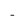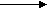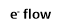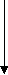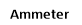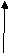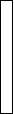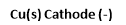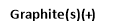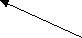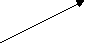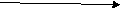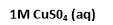Fig 1 Diagram of electrolytic cell used in investigation

In this investigation, a current is passed through the solution with copper as the cathode and graphite as the anode. After a set amount of time, the circuit is disconnected and the mass of the cathode is measured. Following which, calculations are made so as to determine the Avogadro’s and Faraday’s constant.

Qualitative Observations

When the D.C power source was turned on, bubbles were formed at the Graphite anode. As the reaction progresses, powdery substance gets suspended in the solution and a black solid deposits can be found at the bottom of the beaker and there is a visible decomposition of the graphite electrode. As the reaction progress, a pink layer of copper forms on the copper strip. The copper strip is originally brown in colour while the graphite electrode is black in colour. Eventually, as the graphite electrode decomposes, the graphite molecules will turn the copper (II) sulphate solution from blue to black in colour. However, when the copper sulfate solution is filtered, it is noted that there is a decrease in the intensity of the blue colour in the filtrate after the electrolysis. The initial pH of the solution is 2.75, after the electrolysis is carried out, the pH decreases to 2.10.

Data Collection

Constant variables

 Time Interval/s(±0.2) 300 Voltage/V 4V Current/A(±0.01) 0.25

Cathode

 Trial 1 Initial mass/g (± 0.001 g) 1.315 Final mass/g (± 0.001 g) 1.279 Change in mass/g (± 0.002 g) 0.036

Chemical equation for reaction at the anode:

2H2O (l) ïƒ O2 (g) + 4H+ + 4e (aq)

Chemical equation for reaction at the cathode:

Cu2+ (aq) + 2e ïƒ  Cu (s)

Calculations for cathode

 Calculations Error Propagation Change in mass(Cu) = 0.036g Mols of (Cu) =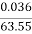=5.7 x 10-4 mol   Number of mol of electrons- Using mol ratio Cu2+ (aq) + 2e– ïƒ  Cu (s) Number of mol of electrons =11.4 x 10-4     Charge flowing through circuit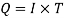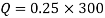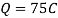Number of electron charges in circut=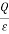Where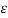is the elementary charge, the charge of one electron Number of electron charges in circuit =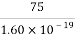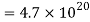Number of mol of electrons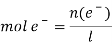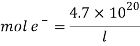Where L is the Avagandro’s constant Equating the number of mols of electrons obtained form the copper mass data and the number of mol of electrons from the current-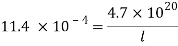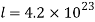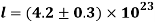Faraday’s constant =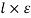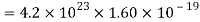= 67000 C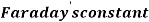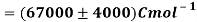%±Δ Mol of Cu =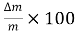%±Δ Mol of Cu =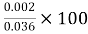= 5.6 % % Uncertainty of number of mol of electrons = % uncertainty of Mass(Cu) =5.6% % uncertainty of number of mol of electrons =5.6% %±Δ charge flowing in circuit =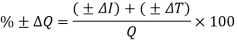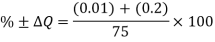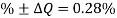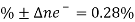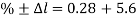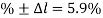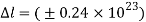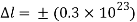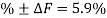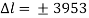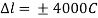Percentage error

Percentage error for Faraday’s Constant =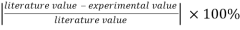=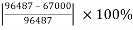= 30%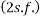Percentage uncertainty of faraday’s constant =5.9%

Percentage systematic error in Faradays’ constant =%error-%random error

= 24.1%

Percentage error for Avogadro’s Constant =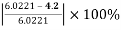= 30%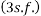Percentage uncertainty of Avogadro’s Constant =5.9%

Percentage systematic error in Avogadro’s Constant =%error-%random error

= 24.1%

Conclusion

In conclusion, the calculated value of Faraday’s constant is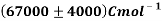mol-1 and Avogadro’s constant is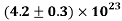.

As seen above, the percentage error for both Faraday’s constant and Avogadro’s constant are both 30% and after subtracting the error due to instrumental uncertainty, the % systematic error obtained is 24.1%. This shows that the experimental values calculated differ greatly from the literature values, indicating that there has been a significant amount of systematic error, which has caused the calculated value to be much different from the literature value. As percentage error of both Faraday’s constant and Avogadro’s constant are much larger than their respective percentage uncertainties, this indicates that the sources of systematic error are significant and cannot be ignored

Evaluation

 Metal passivation-en.w,wikepedia.org/Passivation_(Chemistry). Accessed- 26/2/2014)

View all

## DMCA / Removal Request

If you are the original writer of this essay and no longer wish to have your work published on UKEssays.com then please:

Related Services

Related Lectures